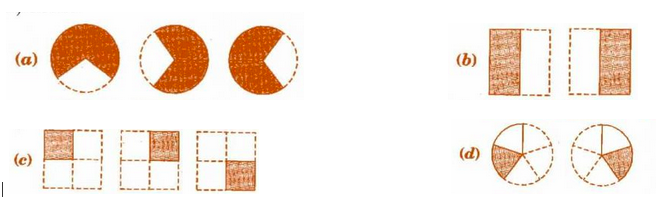# 1.  Which of the drawings (a) to (d) show :P Pankaj Sanodiya

i)

(d) represents two circles with 1 part shaded out of 5 part So, it represents

.

ii) b represents two square one part out of two of both square are shaded, So it represents

(iii)

(a) represents 3 circles 2 parts out of three of all circles are shaded. So it represents

(iv)

(c) represents 3 square with one part out of four-part shaded in each square hence it represents

.

Exams
Articles
Questions